Math Concepts

# How to identify Exponents and Powers?

0

Table of Contents

 1 What are Exponents? 2 Properties of Exponents and Powers 3 Standard Forms of Exponents and Powers 4 Practice Questions 5 Summary 6 About Cuemath 7 Frequently Asked Questions (FAQs) 8 External References

8th January 2021

Reading Time: 4 Minutes

Exponents and Powers are additional operations that go beyond the addition, subtraction, multiplication, and division that have been addressed so far.

Exponential notation is a powerful way to express repeated multiplication of the same number. Specifically, powers of 10 express very large and very small numbers in a manner that is convenient to read, write and compare.

Also read:

## What are Exponents?

Exponents are used to expressing large numbers in the shorter form to make them easy to read, understand, compare and operate upon.

For example: a x a x a x a = a4.

An exponent is a number that is placed to the right of and above another number. So, here it is read as ‘a’ raised to the exponent 4 or the fourth power of ‘a’, where ‘a’ is the base and 4 is the exponent and a4 are called the exponential form. (a x a x a x a) is called the expanded form.

In expression 53, the 3 is called the exponent, and the 5 is called the base. So 53 means a total of three fives multiplied, or 53 = 5 × 5 × 5 = 125. This expression can be read as, “Five to the third power.”

An exponent indicates the number of times to multiply the base number by itself. In simple terms, how many times a particular number is multiplying to itself, is shown by using exponents. Download the PDF below to understand the properties of Exponents and Powers.

 📥 Exponents and Powers Download

## Properties of Exponents and Powers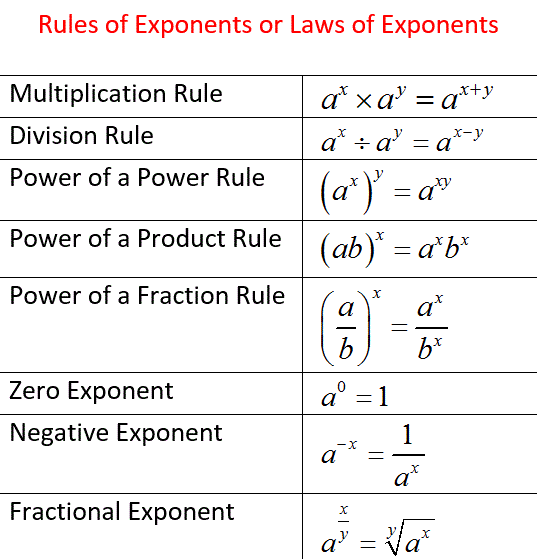1. ### Multiplication Rule

To multiply powers with the same base, keep the base and add the exponents.

Algebraic expression:  ax x ay = ax+y
Example: 35 × 38 = 35+8 = 313

1. ### Division Rule

When you are dividing two powers with the same base, subtract the exponent of the denominator from the exponent of the numerator to give you the exponent of the answer. In other words, to divide powers with the same base, keep the base and subtract the exponents.

Algebraic expression: ax ÷ ay = ax-y
Example: 69 ÷ 64 = 69-4 = 65

1. ### Power of Power Rule

To raise a power to a power, keep the base and multiply the exponents.

Algebraic expression: (ax)y = axy
Example: (94)5 =9(4x5) = 920

1. ### Power of a Product Rule

To simplify the power of a product, find the power of each factor and multiply.

Algebraic expression: (ab)x = ax . bx
Example:  (5 . 2)4 = 54 . 24

1. ### Power of a Fraction Rule

When you raise a fraction to a power you raise both the numerator and the denominator to the same power.

Algebraic expression              Example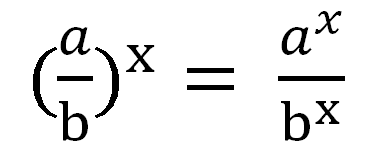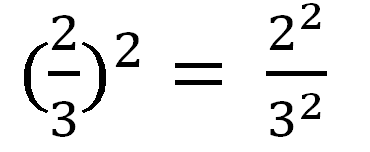1. ### Zero Exponent

Any number that has an exponent of 0 is equal to 1. Any nonzero number with an exponent of 0 is 1, so a0 = 1 when ‘a’ is any number except zero.

Algebraic expression: a0 = 1
Examples: 20 = 1, 30 = 1, 100 = 1

Any number with an exponent of 1 is just the number itself, so a1 = a
Algebraic expression: (a1 = a); For any number a ≠ 0, a0 = 1. You can show this by using the division of powers rule.

If you start with 1000, and keep dividing by 10, you get this pattern

• 1000 = 103

Now divide by 10 : 103 ÷ 101 = 10(3-1) = 10

• 100 = 102

Now divide by 10 : 103 ÷ 101 = 10(2-1) = 101

• 10 = 101

Now divide by 10 : 101 ÷ 101 = 10(1-1) = 10

• 1 = 100
1. ### Negative Exponent

If the exponent is a negative number, it means the reciprocal of the corresponding positive exponent. In simple terms, it means to do the opposite of multiplication which is division.

Algebraic expression             Example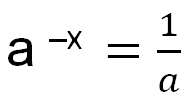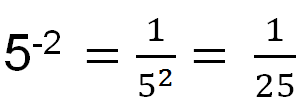1. ### Fractional Exponent

When there is a fractional exponent, the numerator denotes the power and the denominator denotes the root.

Algebraic expression              Example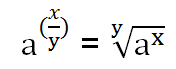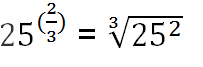A fractional exponent like 25(2/3) means, apply both, a square (2) and a cube root (1/3), in any sequence.

### Squares and Cubes

Two common types of powers are squares and cubes

Squaring a number means to multiply the number by itself: 52 = 5 × 5 = 25. A number like 25 is called a perfect square because it is the square of a whole number. When you cube a number, you raise it to the third power. To do this, simply multiply three of the numbers: 53 = 5 × 5 × 5 = 125. A perfect cube is any whole number cubed.

### Square Roots and Cube Roots

A number can be raised to a power, like 42 = 16 or 23 = 8. This process also can be turned around by asking, “What number squares to give 16?” or “What number would I cube to get 8?” This reverse question is what square and cube roots are about.

## Standard Forms of Exponents and Powers

While working in practical experiences, Astronomers, Biologists, Engineers often come across measuring different quantities, where they are working with either large or small numbers.

If a quantity is written as the product of a power of 10 and a number that is greater than or equal to 1 and less than 10, then the quantity is said to be expressed in standard form (or scientific notation).  It is also called an exponential form.

For example: Here, to express it in shorter form or make it easier to handle, so when a decimal is moved 11 places to the left.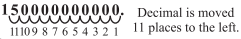Expanded form is 150,000,000,000 = is expressed in a Standard form as 1.5 × 1011 .

## Practice Questions

Question 1: What is the value of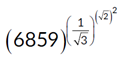(A) 37     (B) 31     (C) 19     (D) 23

Answer:

 (C) 19

Question 2: What is the value of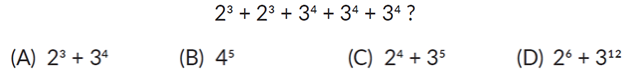Answer:

 (C) 24+35

Question 3: What is the value of n, if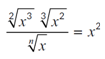for all x > 0?

(A) 6     (B) 2     (C) 5    (D) 3

Answer:

 (A) 6

Question 4: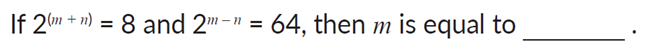(A) 8     (B) 4.5     (C) 2     (D) 16

Answer:

 (B) 4.5

Question 5: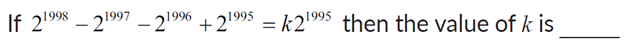(A) 1     (B) 2     (C) 3     (D) 4

Answer:

 (C) 3

Question 6: Earth is 149.7 million km from the sun. Express the distance in standard form.
(A) 1.497 × 109 m

(B) 1.497 × 1011 m

(C) 14.97 × 10m

(D) 149.7 × 106 km

Answer:

 (B) 1.497 × 1011 m

## Summary

• An expression that consists of a repeated power of multiplication of the same factor is called Power/Exponent/Indices.
• Consider an example like 54, the number 5 is called the base, whereas 4 is the power/indices/exponent of the expression.
• The value of the expression is derived by multiplying the base as many times as the number of powers.
• Exponents and powers are used in all technological worlds.
• Exponents and power are seen in use at scientific researches, pH Scale etc.
• Geophysicists use exponents and power to measure the strength of earthquakes, logarithmic scale.

-Written by Dhanshree Kankariya Baldota, Cuemath Teacher

## About Cuemath

Cuemath, a student-friendly mathematics and coding platform, conducts regular Online Live Classes for academics and skill-development, and their Mental Math App, on both iOS and Android, is a one-stop solution for kids to develop multiple skills. Understand the Cuemath Fee structure and sign up for a free trial.

## Are Exponents and Powers the same?

Exponents Powers
Exponents the number of times the base number is used as a factor in multiplying itself It means whole expression depicting the repetition of multiplication of the same number
In 54 = 5×5×5×5=625, the exponent is 4, which denotes the number of times ‘5’ is to be multiplied by itself. In 54 = 5×5×5×5=625, where the base number is ‘5’ which is to be multiplied by itself four times and could be also called as “five to the power of four” or “two to the third power”

## What are the five rules of exponents?

Product of powers rule. When multiplying two bases of the same value, keep the bases the same and then add the exponents together to get the solution. ... Quotient of powers rule. Power of a power rule. Power of a product rule. Power of a quotient rule. Zero power rule. Negative exponent rule.

## External References

To know more about exponents and powers, please visit these blogs:

Related Articles
GIVE YOUR CHILD THE CUEMATH EDGE
Access Personalised Math learning through interactive worksheets, gamified concepts and grade-wise courses
Learn More About Cuemath